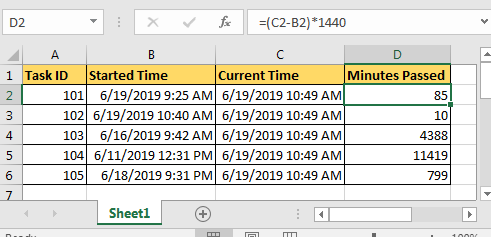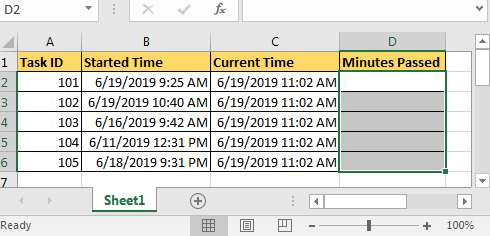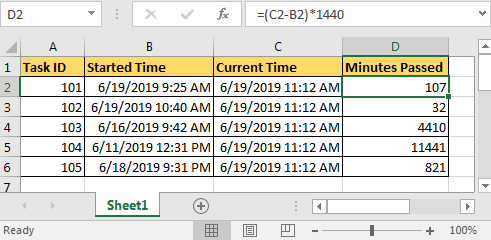# Calculate Minutes Between Date & Time In Microsoft ExcelWhile working with time and dates in excel, you frequently get the need to calculate hours, minutes and seconds between two timestamps.

Well, in excel 2016 calculating the time difference is quite easy. You just need to subtract the start time from the end time.

## Let’s Do It With An Example

You are assigned five tasks. You enter the start time of a task whenever you start it.In the next column you have NOW() function of Excel is running that give. NOW() in excel is used to calculate the current time.

In the next column, you want to calculate the minutes passed since you started that task, as shown in the above image.

The Generic Formula to calculate the minutes between two times is:

 (END TIME - START TIME)*1440

We subtract time/dates in excel to get the number of days. Since a day has 1440 (24*60) minutes, we multiply the result by 1440 to get the exact number of minutes.

For this example, we write the formula below in cell D2 and copy it in the cells below:

 =(C2-B2)*1440So, we cleared our doubts about how to calculate time in excel 2016. This can be done in all versions of Excel including excel 2013, Excel 2010, Excel 2007, and even older versions

Related Articles:

Get days between dates in Excel

Calculate years between dates

How to Add Years to a Date

Calculate Minutes Between Dates & Time In Microsoft Excel

Calculate hours between time in Excel

Multiplying time values and numbers

Calculate age from date of birth

Popular Articles:

50 Excel Shortcut to Increase Your Productivity

The VLOOKUP Function in Excel

COUNTIF in Excel 2016

How to Use SUMIF Function in Excel

1.I have a spreadsheet at work where time worked is simply entered as text for each day. I am trying to take the text and calculate a weekly total for number of hours worked but keep running into errors. It looks like this:

Thursday Friday Saturday Sunday Monday Tuesday Wednesday Total

Jane Doe 5-8 5-8 5-8

In the total column, I want it to do the math for each day (5-8 is three hours per day) so the total would be 9.

I realize the simplest way would be for start and end times to be in different columns and then it is an easy formula, but they want to keep it as is. Can anyone point out what I am not thinking of?

Thanks

2.i am looking for the elegant way to calculate night and day power consumption. the problem is that night tariff is calculated from 23:00 to 7:00, and counter indications are not taken daily.
e.g.
26 October, 13:06
28 October, 11:26
4 November, 17:46
how to calculate the time (hours and minutes) that can be attributed to the night/day time?

3.7 days, 18 hours, 30 minutes and 0 seconds

how to convert this to decimal in excel

4.I'm trying to find the minutes and seconds calculator but I can't find it.

5.this iz smhw great

6."Hi Diane,

I just tried it, and it works perfectly for me in Excel 97 and Excel 2000.

You do not indicate *why* or *how* it doesn't work, but I would gues the most likely cause it that either your dates or times do not meet the criteria I outlined above.

If you clean up the two 'input' columns first, does it then work?

Alan.
DATE WORKSHEET AUTOMATICAL
JEFF Posted on: 31-12-1969
WHEN I OPEN THE WORKSHEET I WANT THE WORKSHEET DATED, BUT WHEN I SAVE THE WORKSHEET I DO NOT WANT IT TO CHANGE THE DATE LATER WHEN I OPEN IT AGIAN."

7."Thank you, Alan, however the tip you shared did not yield the desired outcome.

A B C
1 4/11/2003 07:46
2 4/11/2003 07:46 4/11/03 7:46

I have data as seen in line one, and can not find a formula or method to combine the date & time into ONE column without losing data. In line two here, I show my desired product. End goal is to determine time intervals between different DATES AND TIMES. TQM to the max! desperate!! Anybody???"

8."Hi Diane,

Assuming that your two columns (date and time) are already 'clean' and are in A1:B4 then you can just add them together:

C1 = A1 + B1

However, for this to work, your dates need to be exact dates, not dates with times (in other words the date values are integers), and the times need to be pure times, with no date element (in other words, the time values need to be greater than or equal to zero, and less than 1).

HTH,

Alan."

9.Need to combine date into one column so that other calculation formulas can handle them for subtraction from other dates & times to yield hours and minutes between two dates. Help---diane

10."If you don't see a custom format already listed, just type it into the box above the list.

Alan."

11."Hi Tom,

If you have the time spent in column A, and the units in column B as follows (A1:B4):

1:48 50000
3:06 85000
4:42 113000
0:35 17000

I am assuming that the times are entered as times (not as text for example).

Then you can calculate the average units per day (being 24 hours) as follows in column C:

=B1/A1

You may have to format the answer to General (for example) since it will probably auto-format as a time.

In this example, C1 = 666666.666... units per day (24 hours).

If you want it per hour, then divide by 24.

If you want it per minute then divide by 1440 (24*60).

Just watch your units and you should be fine.

Alan."

12."I don't see the [m] format in my custom formats.
I am still finding it difficult to establish an hourly rate formula when an operator works (for example) 1:48 and produces 50,000 units. I can tell the system to add 60 + 48 to get 108 minutes and then procede, but how can we calculate if there are 3, 4 or 0 hours plus minutes possible? (IE: 3:06 / 4:42 / 0.35)"

13."Search for this tip at this web site:

Add time value exceed 24 hours in Microsoft Excel
"

14."Anyone know the formula in excel for calculating Hrs between start time and end time to come up with hours worked less 30 minutes for lunch and have the results for each employee you add total up in one cel?
ie,
Employee #1 Start Time in B8=9:00 A.M End Time =5:30 P.M. This should = in B7 8 hrs.
Employee #2 Start Time in B9=9:00 A.M End Time =5:30 P.M. This should = in B7 16 hrs.
Employee #3 Start Time in B10=9:00 A.M End Time =5:30 P.M. This should = in B7 24 hrs.
And so on, as I have 35 employees.
Would appreciate your help! Thanks"

15."Anyone know the formula for calculating Hrs between start tme and end time to come up with hours worked less 30 minutes for lunch?
ie, Start Time =9:00 A.M End Time =5:30 P.M. This should = 8 hrs.
Would appreciate your help! Thanks"

16."Hi Damien,

First thing I would check is your units.

Are you recording the time in standard Excel units (being days = 24 hours)?

If not, then when you try to add them togather you may get odd results.

Note that you can format the cells to *show* your time in hours - that is a separate issue from what the cells contain though.

That does not mean you cannot use hours or any other units you like, but if you do, you need to be very careful throughout your calculations.

Alan."

17.I have done a daily time sheet in excel, now when I try to total the Total worked hours for a month, it just does not seem to work.. can u help

18."Hi Craig,

I will assume that you mean that the columns contain date / time values to two decimal places.

If so, subtract one from the other, and this will give you the time difference in days (Excel's native unit of time).

You can then format the rsult to display this answer in minutes by using a custom number format such as:

[m]"" mins""

This will *display* the answer in minutes.

Hope that helps,

Alan."

19."excele
nt useful tips. thank u .
phani kumar"

20.I have 2 columns with a start time and finish time. However, the columns are both in 'number' format (to 2 decimal places). How can I calculate the time difference in minutes? Can anyone help me ?

21."Hi Diane,

I just tried it, and it works perfectly for me in Excel 97 and Excel 2000.

You do not indicate *why* or *how* it doesn't work, but I would gues the most likely cause it that either your dates or times do not meet the criteria I outlined above.

If you clean up the two 'input' columns first, does it then work?

Alan.
DATE WORKSHEET AUTOMATICAL
JEFF Posted on: 31-12-1969
WHEN I OPEN THE WORKSHEET I WANT THE WORKSHEET DATED, BUT WHEN I SAVE THE WORKSHEET I DO NOT WANT IT TO CHANGE THE DATE LATER WHEN I OPEN IT AGIAN.
"

22."Thank you, Alan, however the tip you shared did not yield the desired outcome.

A B C
1 4/11/2003 07:46
2 4/11/2003 07:46 4/11/03 7:46

I have data as seen in line one, and can not find a formula or method to combine the date & time into ONE column without losing data. In line two here, I show my desired product. End goal is to determine time intervals between different DATES AND TIMES. TQM to the max! desperate!! Anybody???"

23."Hi Diane,

Assuming that your two columns (date and time) are already 'clean' and are in A1:B4 then you can just add them together:

C1 = A1 + B1

However, for this to work, your dates need to be exact dates, not dates with times (in other words the date values are integers), and the times need to be pure times, with no date element (in other words, the time values need to be greater than or equal to zero, and less than 1).

HTH,

Alan."

24.Need to combine date into one column so that other calculation formulas can handle them for subtraction from other dates & times to yield hours and minutes between two dates. Help---diane

25."If you don't see a custom format already listed, just type it into the box above the list.

Alan."

26."Hi Tom,

If you have the time spent in column A, and the units in column B as follows (A1:B4):

1:48 50000
3:06 85000
4:42 113000
0:35 17000

I am assuming that the times are entered as times (not as text for example).

Then you can calculate the average units per day (being 24 hours) as follows in column C:

=B1/A1

You may have to format the answer to General (for example) since it will probably auto-format as a time.

In this example, C1 = 666666.666... units per day (24 hours).

If you want it per hour, then divide by 24.

If you want it per minute then divide by 1440 (24*60).

Just watch your units and you should be fine.

Alan. "

27."I don't see the [m] format in my custom formats.
I am still finding it difficult to establish an hourly rate formula when an operator works (for example) 1:48 and produces 50,000 units. I can tell the system to add 60 + 48 to get 108 minutes and then procede, but how can we calculate if there are 3, 4 or 0 hours plus minutes possible? (IE: 3:06 / 4:42 / 0.35)"

28."Search for this tip at this web site:

Add time value exceed 24 hours in Microsoft Excel
"

29."Anyone know the formula in excel for calculating Hrs between start time and end time to come up with hours worked less 30 minutes for lunch and have the results for each employee you add total up in one cel?
ie,
Employee #1 Start Time in B8=9:00 A.M End Time =5:30 P.M. This should = in B7 8 hrs.
Employee #2 Start Time in B9=9:00 A.M End Time =5:30 P.M. This should = in B7 16 hrs.
Employee #3 Start Time in B10=9:00 A.M End Time =5:30 P.M. This should = in B7 24 hrs.
And so on, as I have 35 employees.
Would appreciate your help! Thanks"

30."Anyone know the formula for calculating Hrs between start tme and end time to come up with hours worked less 30 minutes for lunch?
ie, Start Time =9:00 A.M End Time =5:30 P.M. This should = 8 hrs.
Would appreciate your help! Thanks"

31."Hi Damien,

First thing I would check is your units.

Are you recording the time in standard Excel units (being days = 24 hours)?

If not, then when you try to add them togather you may get odd results.

Note that you can format the cells to *show* your time in hours - that is a separate issue from what the cells contain though.

That does not mean you cannot use hours or any other units you like, but if you do, you need to be very careful throughout your calculations.

Alan."

32.I have done a daily time sheet in excel, now when I try to total the Total worked hours for a month, it just does not seem to work.. can u help

33."Hi Craig,

I will assume that you mean that the columns contain date / time values to two decimal places.

If so, subtract one from the other, and this will give you the time difference in days (Excel's native unit of time).

You can then format the rsult to display this answer in minutes by using a custom number format such as:

[m]"" mins""

This will *display* the answer in minutes.

Hope that helps,

Alan."

34."excele
nt useful tips. thank u .
phani kumar"

35.I have 2 columns with a start time and finish time. However, the columns are both in 'number' format (to 2 decimal places). How can I calculate the time difference in minutes? Can anyone help me ?

Terms and Conditions of use

The applications/code on this site are distributed as is and without warranties or liability. In no event shall the owner of the copyrights, or the authors of the applications/code be liable for any loss of profit, any problems or any damage resulting from the use or evaluation of the applications/code.